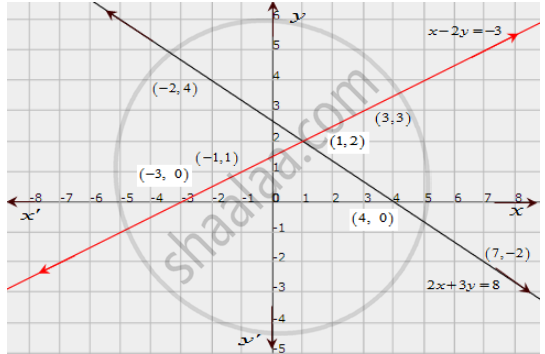# Solve Graphically Each of the Following Systems of Linear Equations. Also, Find the Coordinates of the Points Where the Lines Meet the Axis Of X In Each System. 2x + 3y = 8 X − 2y = −3 - Mathematics

Solve graphically each of the following systems of linear equations. Also, find the coordinates of the points where the lines meet the axis of x in each system.

2x + 3y = 8
x − 2y = −3

#### Solution

The given equations are

2x + 3y = 8 ..........(i)

x − 2y = −3 ..........(ii)

The two points satisfying (i) can be listed in a table as,

 x -2 7 y 4 -2

The two points satisfying (ii) can be listed in a table as,

 x -1 3 y 1 3

Now, the graph of equations (i) and (ii) can be drawn as,It is seen that the solution of the given system of equations is given by x = 1, y = 2.

Also, it is observed that the coordinates of the points where the lines (i) and (ii) meet the x-axis are (4,0) and (3,0) respectively.

Concept: Graphical Method of Solution of a Pair of Linear Equations
Is there an error in this question or solution?

#### APPEARS IN

RD Sharma Class 10 Maths
Chapter 3 Pair of Linear Equations in Two Variables
Exercise 3.2 | Q 29.4 | Page 30